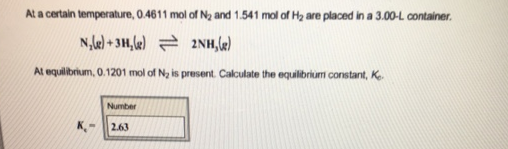# Problem: At a certain temperature, 0.4611 mol of N2 and 1.541 mol of H2 are placed in a 3.00-L container.At equilibrium, 0.1201 mol of N2 is present. Calculate the equilibrium constant, Kc.

###### FREE Expert Solution
97% (320 ratings)###### Problem Details

At a certain temperature, 0.4611 mol of N2 and 1.541 mol of H2 are placed in a 3.00-L container.

At equilibrium, 0.1201 mol of N2 is present. Calculate the equilibrium constant, Kc.